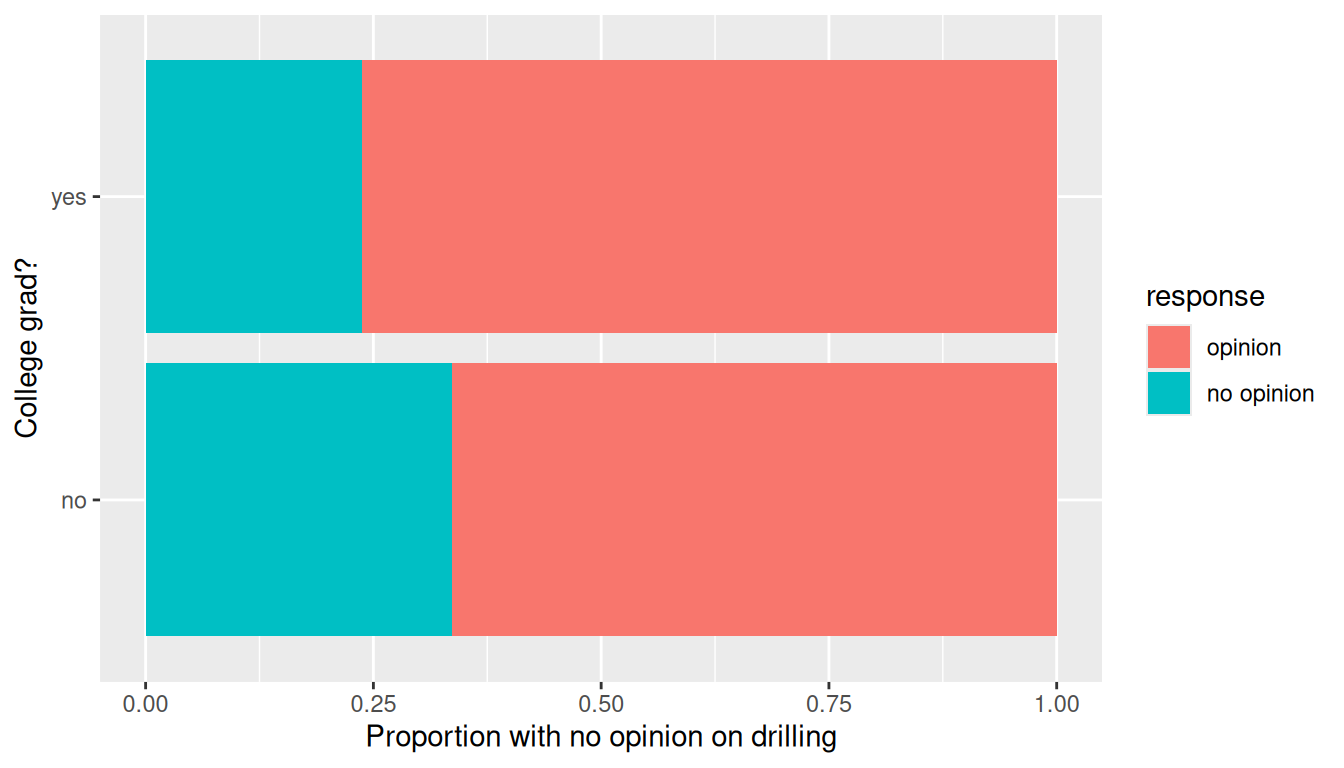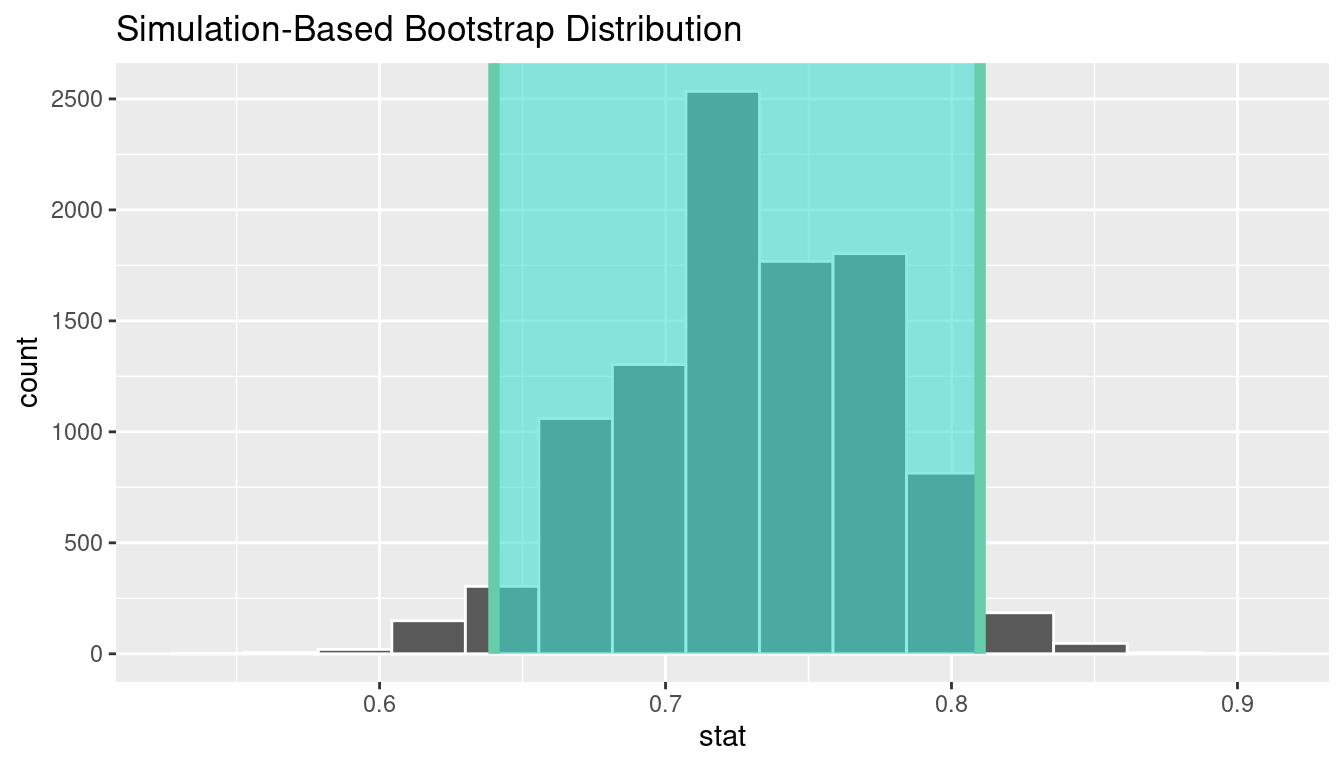## B.4 Two proportions

### B.4.1 Problem statement

A 2010 survey asked 827 randomly sampled registered voters in California “Do you support? Or do you oppose? Drilling for oil and natural gas off the Coast of California? Or do you not know enough to say?” Conduct a hypothesis test to determine if the data provide strong evidence that the proportion of college graduates who do not have an opinion on this issue is different than that of non-college graduates. (Tweaked a bit from Diez, Barr, and Çetinkaya-Rundel 2014 [Chapter 6])

### B.4.2 Competing hypotheses

#### In words

• Null hypothesis: There is no association between having an opinion on drilling and having a college degree for all registered California voters in 2010.

• Alternative hypothesis: There is an association between having an opinion on drilling and having a college degree for all registered California voters in 2010.

#### Another way in words

• Null hypothesis: The probability that a Californian voter in 2010 having no opinion on drilling and is a college graduate is the same as that of a non-college graduate.

• Alternative hypothesis: These parameter probabilities are different.

#### In symbols (with annotations)

• $$H_0: \pi_{college} = \pi_{no\_college}$$ or $$H_0: \pi_{college} - \pi_{no\_college} = 0$$, where $$\pi$$ represents the probability of not having an opinion on drilling.
• $$H_A: \pi_{college} - \pi_{no\_college} \ne 0$$

#### Set $$\alpha$$

It’s important to set the significance level before starting the testing using the data. Let’s set the significance level at 5% here.

### B.4.3 Exploring the sample data

offshore <- read_csv("https://moderndive.com/data/offshore.csv")
counts <- offshore %>% tabyl(college_grad, response)
counts
 college_grad no opinion opinion
no        131     258
yes        104     334

Observe that of the college graduates, a proportion of 104/(104 + 334) = 0.237 have no opinion on drilling. On the other hand, of the non-college graduates, a proportion of 131/(131 + 258) = 0.337 have no opinion on drilling, whereas . The difference in these proportions is 0.237 - 0.337 = -0.099.

Let’s visualize these in a barchart. However, we first reverse the order of the levels in the categorical variable response using the fct_rev() function from the forcats package. We do this because the default ordering of levels in a factor is alphanumeric. However, we are interested in proportions that have no opinion and not opinion. Thus we need to reverse the default alphanumeric order.

offshore <- offshore %>%
mutate(response = fct_rev(response))

ggplot(offshore, aes(x = college_grad, fill = response)) +
geom_bar(position = "fill") +
labs(x = "College grad?", y = "Proportion with no opinion on drilling") +
coord_flip()We are looking to see if a difference exists in the size of the bars corresponding to no opinion for the plot. Based solely on the plot, we have little reason to believe that a difference exists since the bars seem to be about the same size, BUT…it’s important to use statistics to see if that difference is actually statistically significant!

#### Collecting summary info

The observed statistic is

d_hat <- offshore %>%
specify(response ~ college_grad, success = "no opinion") %>%
calculate(stat = "diff in props", order = c("yes", "no"))
d_hat
# A tibble: 1 x 1
stat
<dbl>
1 -0.0993180

#### Randomization for hypothesis test

In order to ascertain if the observed sample proportion with no opinion for college graduates of 0.237 is statistically different than the observed sample proportion with no opinion for non-college graduates of 0.337, we need to account for the sample sizes. Note that this is the same as ascertaining if the observed difference in sample proportions -0.099 is statistically different than 0. We also need to determine a process that replicates how the original group sizes of 389 and 438 were selected.

We can use the idea of randomization testing (also known as permutation testing) to simulate the population from which the sample came (with two groups of different sizes) and then generate samples using shuffling from that simulated population to account for sampling variability.

set.seed(2018)
null_distn_two_props <- offshore %>%
specify(response ~ college_grad, success = "no opinion") %>%
hypothesize(null = "independence") %>%
generate(reps = 10000) %>%
calculate(stat = "diff in props", order = c("yes", "no"))
null_distn_two_props %>% visualize()We can next use this distribution to observe our $$p$$-value. Recall this is a two-tailed test so we will be looking for values that are greater than or equal to -0.099 or less than or equal to 0.099 for our $$p$$-value.

null_distn_two_props %>%
visualize(obs_stat = d_hat, direction = "two_sided")##### Calculate $$p$$-value
pvalue <- null_distn_two_props %>%
get_pvalue(obs_stat = d_hat, direction = "two_sided")
pvalue
# A tibble: 1 x 1
p_value
<dbl>
1 0.00240000

So our $$p$$-value is 0.002 and we reject the null hypothesis at the 5% level. You can also see this from the histogram above that we are far into the tails of the null distribution.

#### Bootstrapping for confidence interval

We can also create a confidence interval for the unknown population parameter $$\pi_{college} - \pi_{no\_college}$$ using our sample data with bootstrapping.

boot_distn_two_props <- offshore %>%
specify(response ~ college_grad, success = "no opinion") %>%
generate(reps = 10000) %>%
calculate(stat = "diff in props", order = c("yes", "no"))
ci <- boot_distn_two_props %>%
get_ci()
ci
# A tibble: 1 x 2
2.5%    97.5%
<dbl>      <dbl>
1 -0.160030 -0.0379112
boot_distn_two_props %>%
visualize(endpoints = ci, direction = "between")We see that 0 is not contained in this confidence interval as a plausible value of $$\pi_{college} - \pi_{no\_college}$$ (the unknown population parameter). This matches with our hypothesis test results of rejecting the null hypothesis. Since zero is not a plausible value of the population parameter, we have evidence that the proportion of college graduates in California with no opinion on drilling is different than that of non-college graduates.

Interpretation: We are 95% confident the true proportion of non-college graduates with no opinion on offshore drilling in California is between 0.16 dollars smaller to 0.04 dollars smaller than for college graduates.

#### B.4.5.1 Check conditions

Remember that in order to use the short-cut (formula-based, theoretical) approach, we need to check that some conditions are met.

1. Independent observations: Each case that was selected must be independent of all the other cases selected.

This condition is met since cases were selected at random to observe.

2. Sample size: The number of pooled successes and pooled failures must be at least 10 for each group.

We need to first figure out the pooled success rate: $\hat{p}_{obs} = \dfrac{131 + 104}{827} = 0.28.$ We now determine expected (pooled) success and failure counts:

$$0.28 \cdot (131 + 258) = 108.92$$, $$0.72 \cdot (131 + 258) = 280.08$$

$$0.28 \cdot (104 + 334) = 122.64$$, $$0.72 \cdot (104 + 334) = 315.36$$

3. Independent selection of samples: The cases are not paired in any meaningful way.

We have no reason to suspect that a college graduate selected would have any relationship to a non-college graduate selected.

### B.4.6 Test statistic

The test statistic is a random variable based on the sample data. Here, we are interested in seeing if our observed difference in sample proportions corresponding to no opinion on drilling ($$\hat{p}_{college, obs} - \hat{p}_{no\_college, obs}$$ = -0.092) is statistically different than 0. Assuming that conditions are met and the null hypothesis is true, we can use the standard normal distribution to standardize the difference in sample proportions ($$\hat{P}_{college} - \hat{P}_{no\_college}$$) using the standard error of $$\hat{P}_{college} - \hat{P}_{no\_college}$$ and the pooled estimate:

$Z =\dfrac{ (\hat{P}_1 - \hat{P}_2) - 0}{\sqrt{\dfrac{\hat{P}(1 - \hat{P})}{n_1} + \dfrac{\hat{P}(1 - \hat{P})}{n_2} }} \sim N(0, 1)$ where $$\hat{P} = \dfrac{\text{total number of successes} }{ \text{total number of cases}}.$$

#### Observed test statistic

While one could compute this observed test statistic by “hand”, the focus here is on the set-up of the problem and in understanding which formula for the test statistic applies. We can use the prop.test function to perform this analysis for us.

z_hat <- offshore %>%
specify(response ~ college_grad, success = "no opinion") %>%
calculate(stat = "z", order = c("yes", "no"))
z_hat
# A tibble: 1 x 1
stat
<dbl>
1 -3.16081

The observed difference in sample proportions is 3.16 standard deviations smaller than 0.

The $$p$$-value—the probability of observing a $$Z$$ value of -3.16 or more extreme in our null distribution—is 0.0016. This can also be calculated in R directly:

2 * pnorm(-3.16, lower.tail = TRUE)
 0.00158

### B.4.7 State conclusion

We, therefore, have sufficient evidence to reject the null hypothesis. Our initial guess that a statistically significant difference did not exist in the proportions of no opinion on offshore drilling between college educated and non-college educated Californians was not validated. We do have evidence to suggest that there is a dependency between college graduation and position on offshore drilling for Californians.

### B.4.8 Comparing results

Observing the bootstrap distribution and the null distribution that were created, it makes quite a bit of sense that the results are so similar for traditional and non-traditional methods in terms of the $$p$$-value and the confidence interval since these distributions look very similar to normal distributions. The conditions were not met since the number of pairs was small, but the sample data was not highly skewed. Using any of the methods whether they are traditional (formula-based) or non-traditional (computational-based) lead to similar results.

### References

Diez, David M, Christopher D Barr, and Mine Çetinkaya-Rundel. 2014. Introductory Statistics with Randomization and Simulation. First. Scotts Valley, CA: CreateSpace Independent Publishing Platform. https://www.openintro.org/stat/textbook.php?stat_book=isrs.